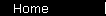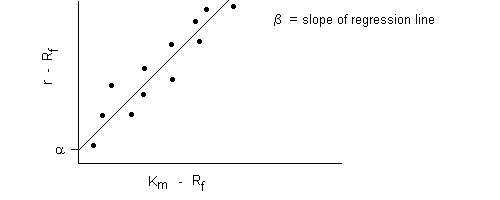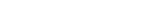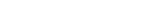### Regression, Alpha, R-Squared

One use of CAPM is to analyze the performance of mutual funds and other portfolios - in particular, to make active fund managers look bad. The technique is to compare the historical risk-adjusted returns (that's the return minus the return of risk-free cash) of the fund against those of an appropriate index, and then use least-squares regression to fit a straight line through the data points:Each data point in this graph shows the risk-adjusted return of the portfolio and that of the index over one time period in the past. (For example, you might make a graph like this with twenty data points, showing the annual returns for each of the past twenty years.)

The general equation of this type of line is

r   -   Rf   =   beta x ( Km - Rf )   +   alpha

where r is the fund's return rate, Rf is the risk-free return rate, and Km is the return of the index.

Note that, except for alpha, this is the equation for CAPM - that is, the beta you get from Sharpe's derivation of equilibrium prices is essentially the same beta you get from doing a least-squares regression against the data. (Also note that alpha and beta are standard symbols that statisticians use all the time for this type of regression; Sharpe and his followers weren't trying to be obscure, as some people like to believe.)

Beta is the slope of this line. Alpha, the vertical intercept, tells you how much better the fund did than CAPM predicted (or maybe more typically, a negative alpha tells you how much worse it did, probably due to high management fees).

The quality of the fit is given by the statistical number r-squared. An r-squared of 1.0 would mean that the model fit the data perfectly, with the line going right through every data point. More realistically, with real data you'd get an r-squared of around .85. From that you would conclude that 85% of the fund's performance is explained by its risk exposure, as measured by beta. (Then you'd punch your fist in the air and say "And the other 15% is due to pure luck!" MPT never believes in investor skill: an investment's behavior equals that of its asset class, minus management fees, plus-or-minus unpredictable luck.)

Next: three factor regression.MPT IntroductionVolatility and TimeEfficient FrontierSharpe RatioBuild a PortfolioIndex InvestingCAPM, BetaAlpha, R-SquaredThree Factor ModelInsurance AnalogyConclusionsBooks & LinksIndex Funds ArticleCAPM CalculatorMarket Simulator

 home  |  article  |  glossary  |  calculator  |  about us  |  books